# Chapter 7: Big O Analysis of Algorithms

• 比喻
• 大 O 复杂度时间
• 最好情况、最坏情况和预期情况
• 大 O 示例

# Big O complexity time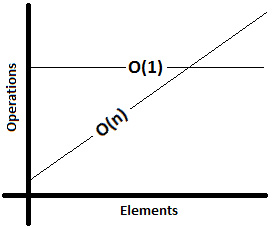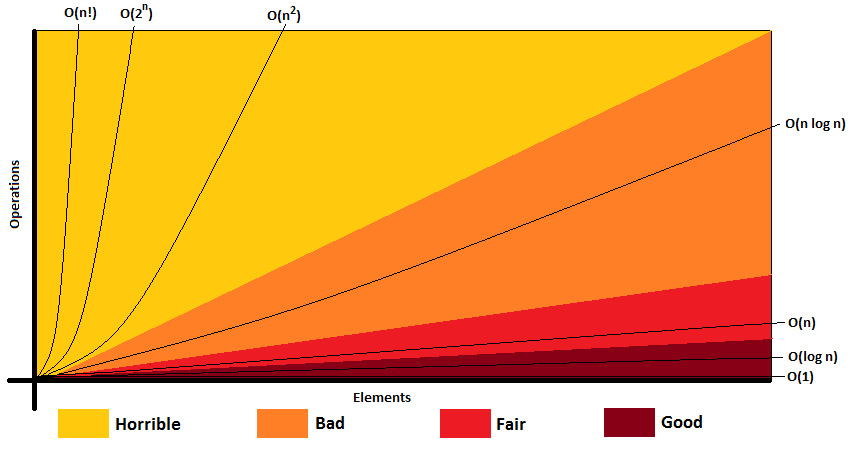# Big O examples

• 丢弃常数
• 删除非主导项
• 不同的输入意味着不同的变量
• 不同的步骤相加或相乘

## Example 1 – O(1)

``````// snippet 1
return 23;``````

``````// snippet 2 - 'cars' is an array
int thirdCar = cars;``````

``````// snippet 3 - 'cars' is a 'java.util.Queue'
Car car = cars.peek();``````

`Queue#peek()` 方法检索但不删除此队列的头部（第一个元素）。头部后面有多少元素并不重要，通过 `peek()` 方法检索头部的时间是 O(1)。

## Example 2 – O(n), linear time algorithms

``````// snippet 1 - 'a' is an array
for (int i = 0; i < a.length; i++) {
System.out.println(a[i]);
}``````

## Example 3 – O(n), dropping the constants

``````// snippet 1 - 'a' is an array
for (int i = 0; i < a.length; i++) {
System.out.println("Current element:");
System.out.println(a[i]);
System.out.println("Current element + 1:");
System.out.println(a[i] + 1);
}``````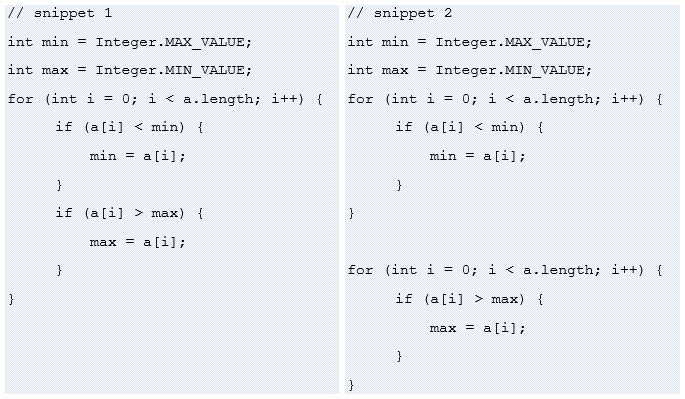7.3 – 代码比较

Big O 并不是要计算代码语句。它的目标是表达输入大小的运行时增长并表达运行时如何扩展。简而言之，Big O 只是描述了运行时的增长率。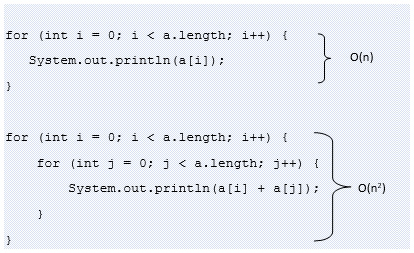7.4 – 在 O(n) 中执行的代码片段

• O(2n + 2n) ->丢弃常数和非显性项-> O(2n)。
• O(n + log n) ->删除非主导词->上）。
• O(3*n2 + n + 2*n) -> drop constants and non-dominant terms -> O(n2).

重要的提示

根据经验，当您表达 Big O 时，请删除非主导项。7.5 – 代码片段 1 和 2

## Example 6 – different steps are summed or multiplied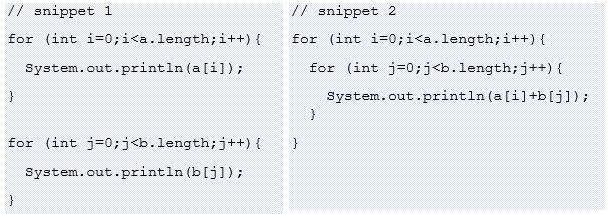7.6 – 代码片段 a 和 b

## Example 7 – log n runtimes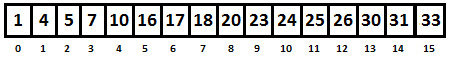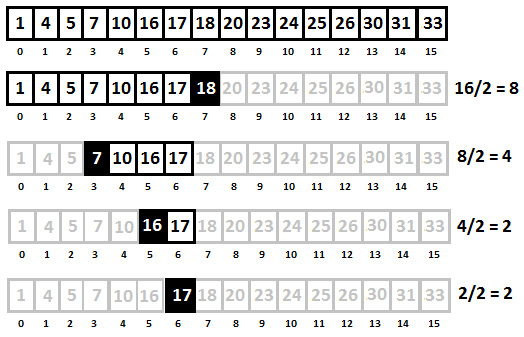``````search 17 in {1, 4, 5, 7, 10, 16, 17, 18, 20,
23, 24, 25, 26, 30, 31, 33}
compare 17 to 18 -> 17 < 18
search 17 in {1, 4, 5, 7, 10, 16, 17, 18}
compare 17 to 7 -> 17 > 7
search 17 in {7, 10, 16, 17}
compare 17 to 16 -> 17 > 16
search 17 in {16, 17}
compare 17 to 17
return``````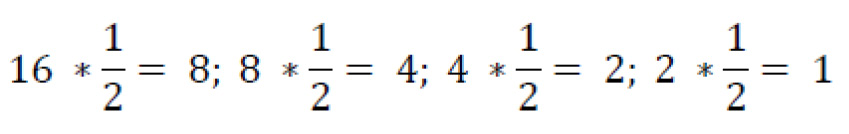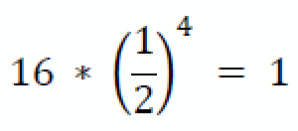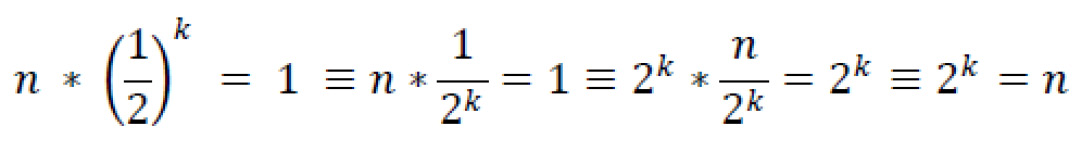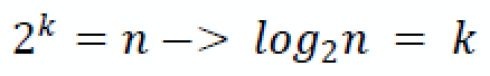``````int fibonacci(int k) {
if (k <= 1) {
return k;
}
return fibonacci(k - 2) + fibonacci(k - 1);
}``````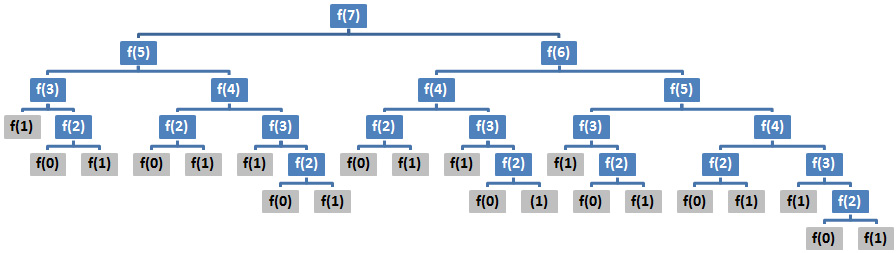## Example 9 – in-order traversal of a binary tree

``````void printInOrder(Node node) {
if (node != null) {
printInOrder(node.left);
System.out.print(" " + node.element);
printInOrder(node.right);
}
}``````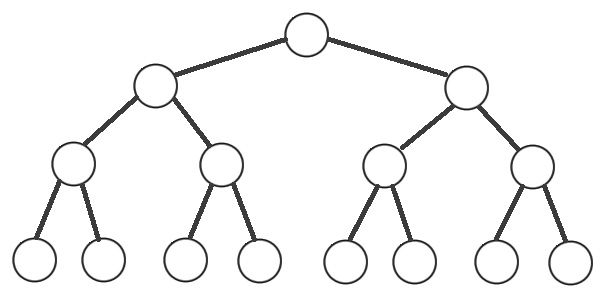• 对于 15 个节点，我们的深度为 4；因此，我们有 24 = 16，相当于 log216 = 4。
• 对于 7 个节点，我们的深度为 3；因此，我们有 23 = 8，相当于 log28 = 3。
• 对于 31 个节点，我们的深度为 5；因此，我们有 25 = 32，相当于 log232 = 5。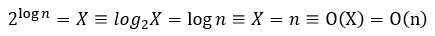## Example 10 – n may vary

``````void printFibonacci(int k) {
for (int i = 0; i < k; i++) {
System.out.println(i + ": " + fibonacci(i));
}
}
int fibonacci(int k) {
if (k <= 1) {
return k;
}
return fibonacci(k - 2) + fibonacci(k - 1);
}``````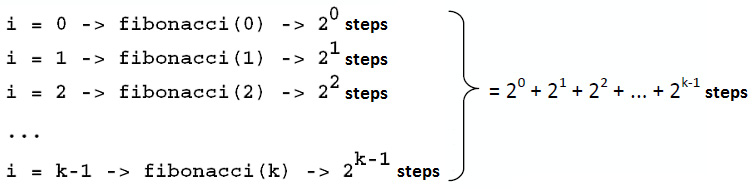## Example 11 – memoization

``````void printFibonacci(int k) {
int[] cache = new int[k];
for (int i = 0; i < k; i++) {
System.out.println(i + ": " + fibonacci(i, cache));
}
}
int fibonacci(int k, int[] cache) {
if (k <= 1) {
return k;
} else if (cache[k] > 0) {
return cache[k];
}
cache[k] = fibonacci(k - 2, cache)
+ fibonacci(k - 1, cache);
return cache[k];
}``````

``````Calling fibonacci(0):
Result of fibonacci(0) is 0
Calling fibonacci(1):
Result of fibonacci(1) is 1
Calling fibonacci(2):
fibonacci(0)
fibonacci(1)
fibonacci(2) is computed and cached at cache
Result of fibonacci(2) is 1
Calling fibonacci(3):
fibonacci(1)
fibonacci(2) is fetched from cache as: 1
fibonacci(3) is computed and cached at cache
Result of fibonacci(3) is 2
Calling fibonacci(4):
fibonacci(2) is fetched from cache as: 1
fibonacci(3) is fetched from cache as: 2
fibonacci(4) is computed and cached at cache
Result of fibonacci(4) is 3
Calling fibonacci(5):
fibonacci(3) is fetched from cache as: 2
fibonacci(4) is fetched from cache as: 3
fibonacci(5) is computed and cached at cache
Result of fibonacci(5) is 5
Calling fibonacci(6):
fibonacci(4) is fetched from cache as: 3
fibonacci(5) is fetched from cache as: 5
fibonacci(6) is computed and cached at cache
Result of fibonacci(6) is 8``````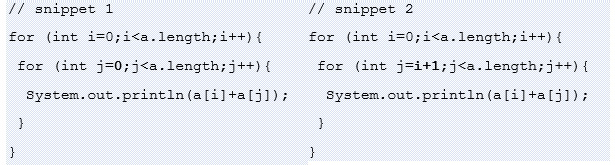7.12 – Big O 的代码片段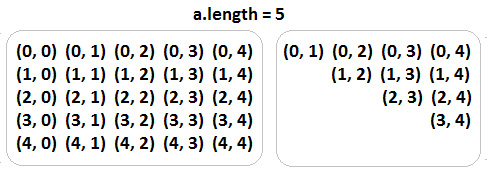• 片段 1 运行时为：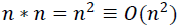• Snippet 2 运行时是：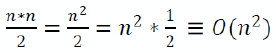因为我们消除了常量。

• 对于第一个片段，内部循环不起作用，它由外部循环运行 n 次，因此 n*n = n 2，结果为 O(n2)。
• 对于第二个片段，内部循环大致完成 n/2 次工作，并且它由外部循环运行 n 次，所以n*n/2 = n2/2 = n2 * 1/2，这导致（删除常量后）O(n2< /跨度>）。

## Example 13 – identifying O(1) loops

``````for (int i = 0; i < a.length; i++) {
for (int j = 0; j < a.length; j++) {
for (int q = 0; q < 1_000_000; q++) {
System.out.println(a[i] + a[j]);
}
}
}``````

``````for (int i = 0; i < a.length; i++) {
for (int j = 0; j < a.length; j++) {
// O(1)
}
}``````

## Example 14 – looping half of the array

``````for (int i = 0; i < a.length / 2; i++) {
System.out.println(a[i]);
}``````

• O(n + p)
• O(n + log n)

## Example 16 – looping with O(log n)

``````for (int i = 0; i < a.length; i++) {
for (int j = a.length; j > 0; j /= 2) {
System.out.println(a[i] + ", " + j);
}
}``````## Example 17 – string comparison

``````String[] sortArrayOfString(String[] a) {
for (int i = 0; i < a.length; i++) {
// sort each string via O(n log n) algorithm
}
// sort the array itself via O(n log n) algorithm
return a;
}``````

`sortArrayOfString()` 接收 `String` 数组并执行两个主要操作。它对该数组和数组本身的每个字符串进行排序。这两种排序都是通过运行时间表示为 O(n log n) 的算法完成的。

• s：最长`String`的长度。
• p`String`数组的大小。

## Example 18 – factorial Big O

``````long factorial(int num) {
if (num >= 1) {
return num * factorial(num - 1);
} else {
return 1;
}
}``````

## Example 19 – using n notation with caution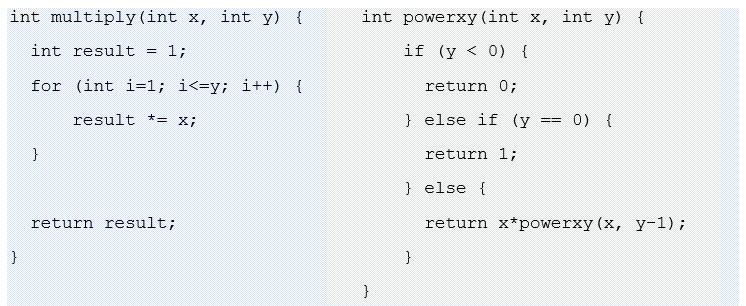7.15 – 代码片段

``````int div(int x, int y) {
int count = 0;
int sum = y;
while (sum <= x) {
sum += y;
count++;
}
return count;
}``````

## Example 21 – the number of iteration counts in Big O

``````int sqrt(int n) {
for (int guess = 1; guess * guess <= n; guess++) {
if (guess * guess == n) {
return guess;
}
}
return -1;
}``````

## Example 22 – digits

``````int sumDigits(int n) {
int result = 0;
while (n > 0) {
result += n % 10;
n /= 10;
}
return result;
}``````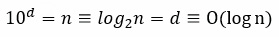## Example 23 – sorting

``````boolean matching(int[] x, int[] y) {
mergesort(y);
for (int i : x) {
if (binarySearch(y, i) >= 0) {
return true;
}
}
return false;
}``````

Example 16 中，我们说过很多排序算法（包括 Merge Sort）的运行时间为 O(n log n)。这意味着 `mergesort(y)` 的运行时间为 O(y log y)。

Example 7中，我们说二分搜索算法的运行时间为 O(log n)。这意味着 `binarySearch(y, i)` 的运行时间为 O(log y)。在最坏的情况下，`for` 循环将迭代整个 `x` 数组，因此将执行二分查找算法`x.length` 次。 `for` 循环的运行时间为 O(x log y)。

# Key hints to look for in an interview

• 如果算法做常数工作，那么大O就是O(1)：这种例子使用输入来执行常数工作（例如，取三个整数，`x``y``w`，并做一些计算，如`x-y``y*w`)。在某些情况下，为了造成混淆，它还会添加重复语句（例如，计算是在 `for(int i=0; i<10; i++)` 中完成的）。因此，从一开始就确定算法的输入是否影响其运行时间非常重要。
• 如果算法循环整个数组或列表，那么总的Big O值可能涉及O(n)：通常，代码片段包含一个或多个循环的重复语句整个输入，通常是一个数组或列表（例如，```for(int i=0; i ，其中 a 是一个数组)。通常，这些结构的运行时间为 O(n)。在某些情况下，为了造成混淆，重复结构会添加一个条件来验证 break 语句。请记住，Big O 是关于最坏情况的情况，因此您应该评估运行时，记住验证 break 语句的条件可能永远不会发生并且 Big O 仍然是 O (n)。 ```
• 如果在每次迭代中，算法将输入数据减半，那么 O(log n) 可能涉及到总的 Big O 值：正如您在 例 7，二分搜索算法是一个 O(log n) 的著名案例。通常，您可以通过尝试可视化运行时来识别类似情况。
• 具有分支的递归问题是一个很好的信号，表明 O(branches depth)总大 O 值的一部分：遇到 O(2depth) 的最常见情况是在操作二叉树的代码片段中。还要注意如何确定 depth。正如您在 Example 9 中看到的，depth 会影响最终结果。在这种情况下，O(2log n) 减少到 O(n)。
• 使用记忆法或制表法的递归算法非常适合将 O(n) 作为其总 Big O 值：通常，递归算法会暴露指数运行时间（例如，O(2< span class="superscript">n))，但 MemoizationTabulation 等优化可能会将运行时间缩短到上）。
• 排序算法通常会在总 Big O 值中引入 O(n log n)：请记住，很多排序算法（例如，堆排序、合并排序等） on) 的运行时间为 O(n log n)。